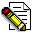## Firefly and PC GAMESS-related discussion clubLearn how to ask questions correctly

problematic SCF convergence

Masoud Nahali
masoudnahali@gmail.com

Dear Firefly Users

My system has passed 23 optimization steps and suddenly at 24th step I have encountered a problematic SCF convergence. As I checked there is not any large spin contamination and the spin parameters are SPIN SZ=2.000
and S-SQUARED=6.289. The system contains Ni,C,O, and H and its multiplicity is 5. Below is my input file parameters and attached please find the SCF iterations. I appreciate your help in advance to find the reason and solve the problem.

\$CONTRL SCFTYP=UHF RUNTYP=OPTIMIZE DFTTYP=B3LYP ECP=read MAXIT=350 MULT=5
ICHARG=0 COORD=unique d5=.t. NZVAR=366 \$END
\$SYSTEM TIMLIM=600000 MWORDS=200 \$END
\$SCF DIRSCF=.TRUE. NCONV=5 \$END
\$STATPT OPTTOL=0.0001 NSTEP=150 \$END
\$zmat dlc=.true. auto=.true. NONVDW(1)=123,4,123,9,123,14,123,1,
123,17,123,8,124,4,124,9,124,16,124,6,124,15,124,10 \$end
! to activate P2P inteface and DLB:
\$P2P P2P=.T. DLB=.T. \$END
! to speed up Huckel guess:
\$GUESS guess=HUCKEL \$END
\$smp call64=.t. mkl64=8 load=0 ssg32=0 msu32=0 mkllvl=3 \$end
\$BASIS gbasis=LANL2DZ extfil=.t. \$END

Best Wishes

Masoud
--------------
Masoud Nahali, Sharif University of TechnologyThis message contains the 26 kb attachment [ A_1.txt ]Sat Mar 26 '11 5:26pmThis message read 812 times